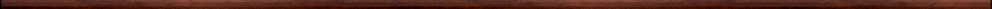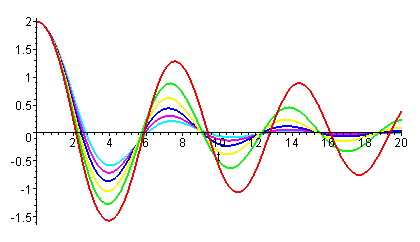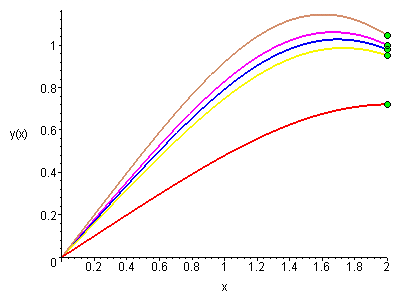Maple worksheets on the numerical solution of 2nd order differential equationsNumerical methods topics:

They are all compatible with Classic Worksheet Maple 10.Taylor series method for 2nd order differential equations - ord2tay.mws

• Description of the Taylor series method for 2nd order differential equations.
• A procedure which can be used to implement the Taylor series method: desolveT2.
The output can be a list of points, or a procedure which uses local Taylor series to interpolate between the fixed points along the approximate solution curve.
An option to use adaptive step-size control is available, which constructs a more efficient and reliable local Taylor series approximation for the solution.

Runge-Kutta methods for 2nd order differential equations - ord2RK.mws

• The procedure desolveK2 can be used as a general "work-horse" for solving linear and certain non-linear 2nd order differential equations numerically. 4-5 order and 7-8 order methods are available. There is an option to see how the the underlying discrete solution is constructed. The output of both methods is in the form of a procedure which can be evaluated throughout a predetermined interval.
The derivative of the solution can also be obtained in either a discrete or continuous form.

Examples involving the numerical solution of 2nd order differential equations - mechRK.mws

• The simple pendulum
• Damped pendulum
• Forced damped pendulum

Examples involving the numerical solution of 2nd order differential equations - mech2RK.mws

• A bead sliding along a wire
• A spring with non-linear damping
• Hard springs

Examples involving the numerical solution of 2nd order differential equations - elecRK.mws

• The response of an RLC circuit to different input voltage functions
• Van der Pol's equation for a non-linear oscillator

More examples involving the numerical solution of 2nd order differential equations - moreRK.mws

• Comparison of numerical solutions given by dsolve and desolveK2.
• Back-tracking
• The Legendre equation

The shooting method for two point boundary value problems - linshoot.mws

• The two point boundary value problem
• Introduction to the shooting method
• The linear shooting method
• Linear shooting method examples

Linear shooting method examples via a procedure - desolveSH.mws

• A procedure for solving two point boundary value problems by shooting methods - desolveSH.
• Linear shooting method examples using desolveSH.

Non-linear shooting method examples - moreSH.mws

• An example of solving a non-linear two point boundary value problem by a shooting method.
• Non-linear shooting method examples using desolveSH.

A procedure for solving first and second order differential equations - desolve.mws

• An interface for the various desolveXX procedures - desolve.
Note: The procedure desolve solves first and second order differential equations of the types usually encountered in an introductory course on differential equations.
Both analytical and numerical solutions can be constructed, and with the option "info=true", the procedure provides step by step information concerning the construction of the solution.
• Order 1 examples
• Order 2 examples

More boundary value problems - genBV.mws

• An example to illustrate the possible non-uniqueness of solutions to boundary value problems.
• Examples

Procedures for differential equations - DEsol.zipTop of page

Main index